# Module 3: Waves and Thermodynamics

In this article, we will go through wave properties, wave behaviour, sound waves and the ray model of light.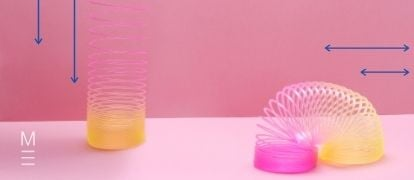Do you need a quick crash course on the Year 11 Module 3: Waves and Thermodynamics? Well, in this article, we explain and break down every topic you will go through in Waves and Thermodynamics!

## Why is this module important?

Throughout all branches of physics, we find some underlying similarities in the way a huge variety of systems behave.

For example, even though we experience sound and light very differently, we can describe both as a wave – a disturbance that transports energy, not matter, as it travels.

By understanding what all waves have in common as well as how to classify them, we are able to investigate a wide variety of phenomena.

This module also covers thermodynamics, the science of thermal energy, temperature and heat. We will learn how to explain real-life examples of heat transfer and temperature changes within a consistent physical framework.

## Need help acing your Physics exam?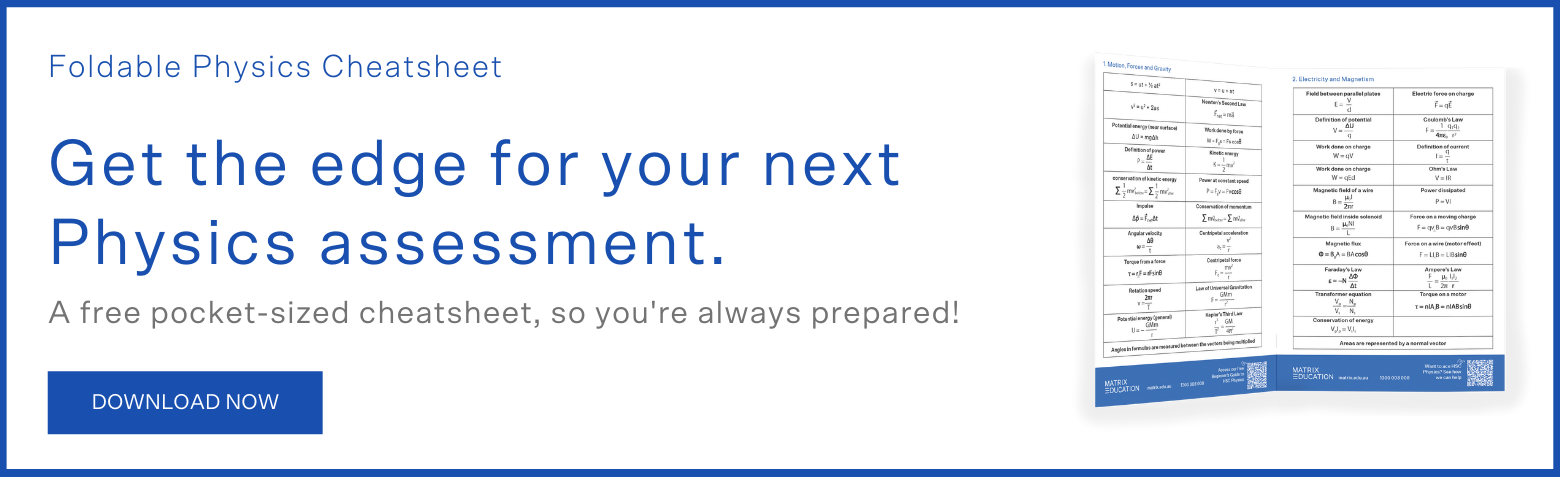## Topic 1: Wave properties

Every wave starts with some sort of disturbance in a medium, followed by the propagation of energy.

For example: a pebble falls into a lake and a ripple spreads outwards from the point of impact.

Another example: a violinist plays a note with her bow and the string begins to vibrate.

Whichever type of wave we observe, we see the transfer of energy but not matter.

In the example with the lake, there is no net flow of water outwards.

The violin string does not travel in any direction. Instead, oscillations spread through the particles of the medium.

(There is one notable exception: electromagnetic waves, a category that includes light and radio waves, do not require a medium to travel. You will investigate this further in Year 12 during Module 7: The Nature of Light.)

We categorise waves in two main ways. The first is the number of dimensions in which the energy travels:

• A one-dimensional wave travels in a straight line, such as a wave on a string.
• A two-dimensional wave spreads out over a plane, such as a water wave or oscillations of a drum head.
• A three-dimensional wave propagates outwards in all directions, such as light being emitted from a bulb.

The other feature we consider is the direction of particle oscillation. A longitudinal wave is carried by oscillations parallel to the wave’s direction of travel.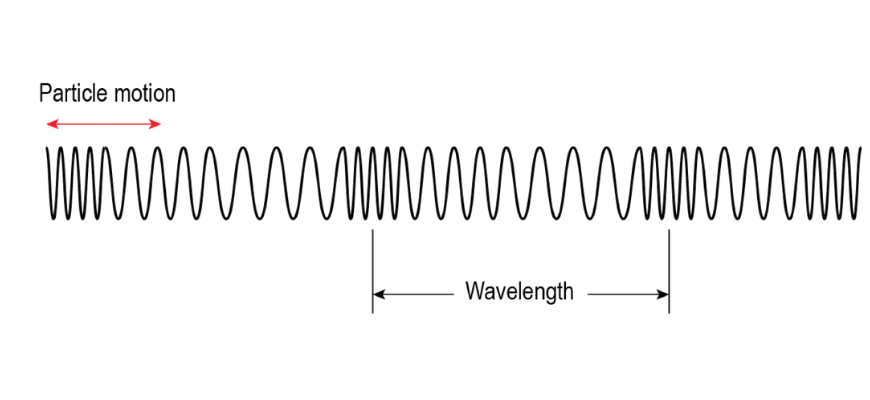By contrast, a transverse wave exhibits oscillations perpendicular to the wave’s directions of travel.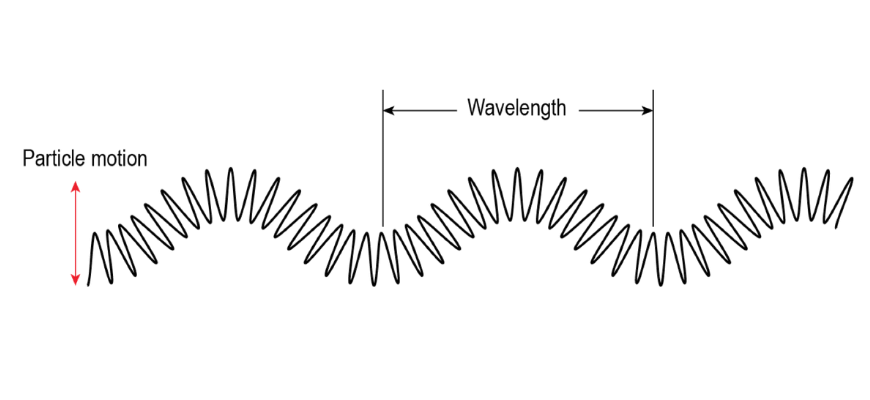## Shoot ahead in Physics with Matrix now!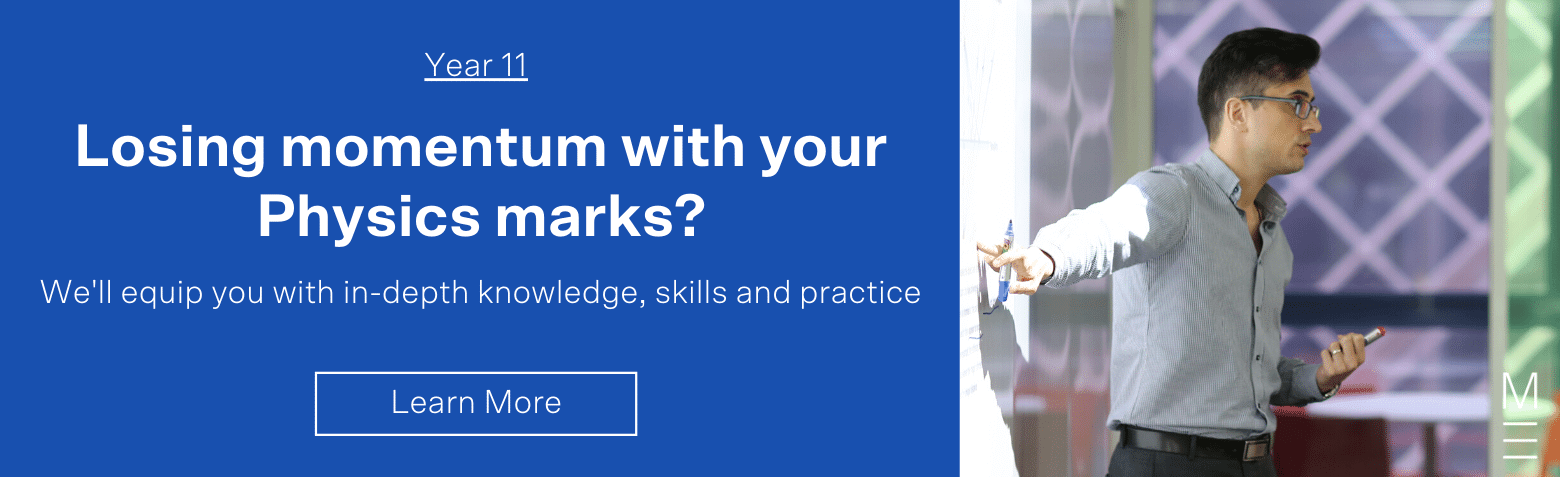## Topic 2: Wave behaviour

The advantage of the wave model is that all waves, regardless of classification, obey the same laws describing their behaviour.

All waves are capable of:

• Reflection
• Refraction
• Dispersion
• Diffraction
• Interference

### Reflection

Reflection occurs when a wave bounces off the boundary where two different media meet.

It is governed by the law of reflection:

$$\text{ angle of incidence } \theta_{1} \ = \text{angle of reflection } \theta_{r}$$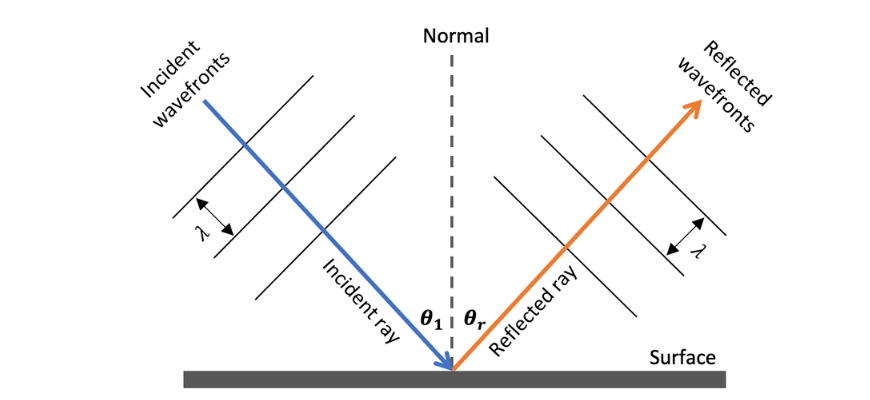### Refraction

In some cases, the wave will enter the second medium, speeding up or slowing down in the process.

When this occurs we observe refraction, an apparent bending of the wave at the boundary.

The angles are related to the wave velocities by Snell’s Law:

\begin{align*}
\frac{v_{1}}{v_{2}} \ = \ \frac{sin \theta _{1}}{sin \theta _{2}}
\end{align*}

Note that the angles are measured relative to the normal.

Reflection and refraction are most obvious in the context of light waves, but they can be observed for every other type of wave.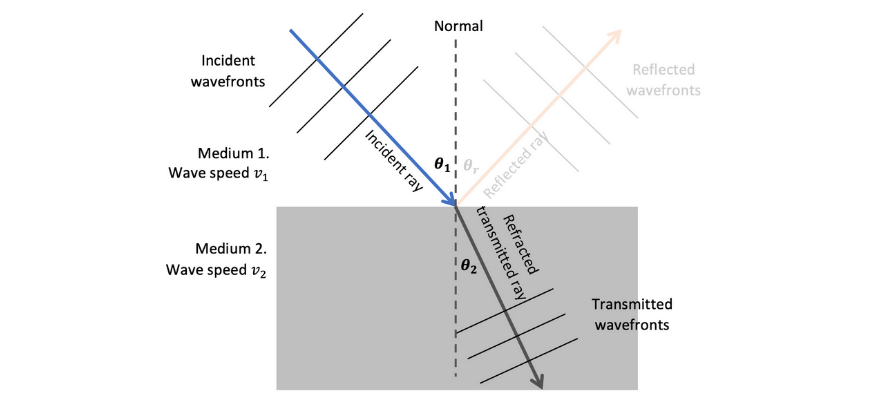### Diffraction and dispersion

Diffraction describes the spreading of waves through and around an obstacle.

Dispersion refers to the phenomenon where the speed of the wave in a medium depends on wavelength.

These are more difficult to express quantitatively, and we will not go into much depth in this course.

### Interference

Finally, interference is what occurs when two waves pass through the same region of space.

The displacements of each wave add together.

If the resulting wave has a higher amplitude, we can refer to the process as constructive interference, but if the amplitudes cancel out we have destructive interference.

## Topic 3: Sound waves

Sound is a longitudinal wave.

It can be produced by the motion of air through pipes, as in wind instruments and the human voice, or by the vibrations of strings on a stringed instrument.

The wave nature of sound is most easily observed using a microphone and oscilloscope, which converts the motion of the air into electrical signals, displaying the resulting waveform on the screen.

### Amplitude and frequency

When you hear something, it is because vibrations have travelled through the air from the source to your ear.

What you hear depends on the amplitude and frequency of the wave: higher amplitudes correspond to louder sounds and higher frequencies correspond to higher pitches.

The average person can hear sounds ranging from a low of 20 Hz to a high of 20 kHz in frequency.

### Three dimensions

A sound wave spreads out in three dimensions. The intensity decreases with the inverse square of the distance to the source; that is,

\begin{align*}
I \ \propto \ \frac{1}{d^{2}}
\end{align*}

due to the power spreading out over a sphere.

### Doppler effect

The Doppler effect is a phenomenon that occurs for all waves, wherein the frequency detected by an observer $$f’$$ depends the frequency of the wave $$f$$ but also on both the observer’s and the source’s motion, but it is most known for its effects on sound.

\begin{align*}
f’ \ = \ f \frac{(v_{wave} \ + \ v_{observer} )}{v_{wave} \ – \ v_{source}}
\end{align*}

This effect explains why car engines, sirens and other sounds produced by fast-moving objects appear to change pitch as the source first approaches and then moves away.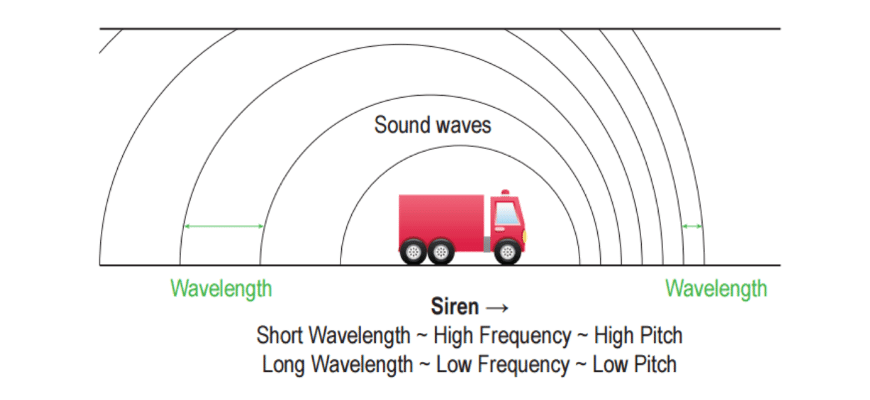## Topic 4: Ray model of light

Light is an electromagnetic wave: it consists of electric and magnetic fields oscillating perpendicular to one another and perpendicular to the direction the light is travelling.

You will learn more about these fields in Module 4: Electricity and Magnetism and again in Module 7: The Nature of Light.

Fortunately for us, we can predict the behaviour of light without a full analysis of the fields involved.

We will treat it as a ray travelling in a straight line and consider what happens when it encounters a change in medium, such as the surface of a mirror (where it will reflect) or a lens (where it will refract).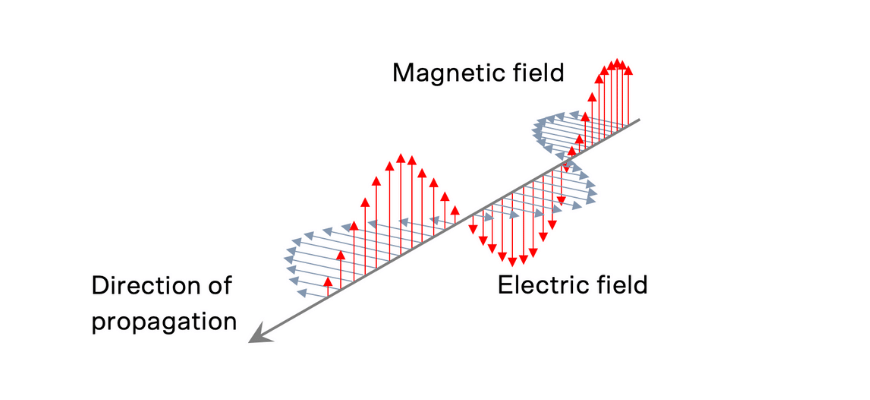### Reflection and refraction

Mirrors and lenses change the paths of light rays to form an image; the light appears to originate from a different place than it does in reality.

This is why objects appear to exist in a space behind mirrors, and how a pair of glasses can make it easier for your eyes to observe distant or nearby things.

### Ray tracing

In the process of ray tracing, we consider three rays of light leaving some part of the object and use the laws of reflection and refraction to predict what happens to the rays.

When an object is viewed in a plane (flat) mirror, we see its image “behind” the mirror. This is because the reflected rays appear to emerge from a point on the other side of the reflective surface.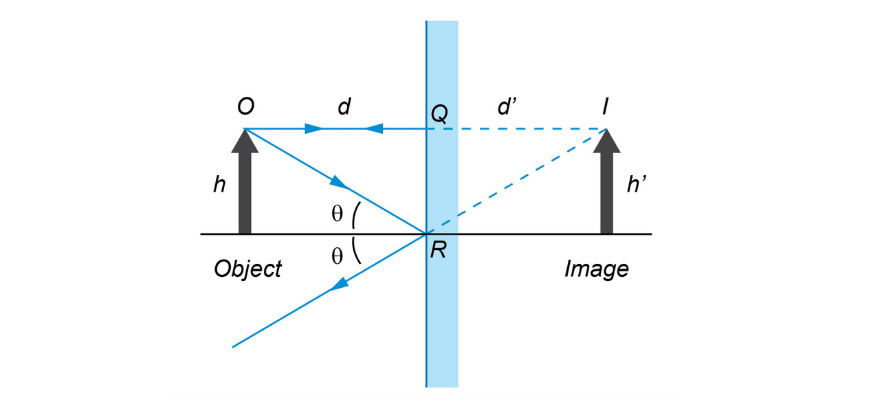You may also need to consider images formed by curved mirrors and lenses in similar ways.

## Topic 5: Thermodynamics

The final topic is short and separate from the previous four. It appears in this module due to space constraints in the syllabus!

### Internal energy

Atoms and molecules possess internal energy, made up of the kinetic energy due to their random motions as well as some internal potential energy.

An object’s total internal energy can change due to the addition or removal of heat.

Absorbing heat will increase the internal energy; losing heat will decrease the internal energy.

As always, energy must be conserved; the heat gained by one object must equal the heat lost by another.

### Addition, removal and transfer of heat

The addition or removal of heat is usually associated with a rise in temperature, such that

\begin{align*}
Q \ = \ mc \Delta T
\end{align*}

where where

• $$Q$$ is the quantity of heat transferred $$(\text{J})$$
• $$m$$ is the mass of the substance $$(\text{kg})$$
• $$\Delta T \ = \ T_{final} \ – \ T_{initial}$$ is the change in the substance’s temperature $$(\text{K})$$
• $$c$$ is the substance’s specific heat capacity $$(\text{Jkg}^{-1}\text{K}^{-1})$$

Another possibility is that the transfer of heat leads to a phase change such as melting or boiling, during which the temperature remains constant. In this scenario,

\begin{align*}
Q_{transitions} \ = \ mL
\end{align*}

### Rate of heat flow

Finally, we can calculate the rate of heat flow (at least when it occurs via conduction, rather than convection or radiation).

It depends on several factors, including the materials involved and the geometry.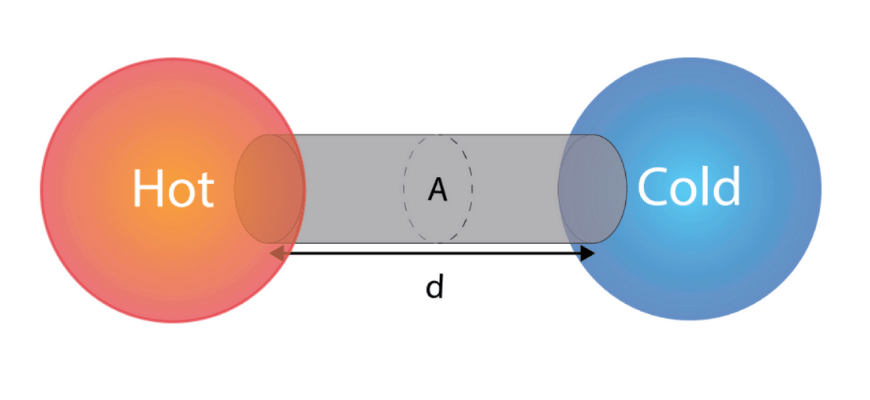The rate of heat transfer between a hot object and a cold object in contact via a patch or conduit of some material is given by

\begin{align*}
P \ = \ \frac{Q}{t} \ = \ \frac{kA \Delta T}{s}
\end{align*}

where:

• $$Q/t$$ is the rate of heat transfer between the objects $$(\text{Js}^{-1} = W)$$
• $$Q/t$$ is a type of power $$P$$
• $$A$$ is the cross sectional area $$(\text{m}^{2})$$
• $$\Delta T$$ is the temperature difference between the two objects $$(\text{K}$$ or $$\text{°C})$$
• $$d$$ is the distance between the two objects (i.e. length of the conduit or thickness of the contact patch), $$(\text{m})$$
• $$k$$ is the thermal conductivity of a material $$(\text{Wm}^{-1}\text{K}^{-1})$$

## Looking ahead to Year 12

In Year 12, you will explore electromagnetic waves in more detail. Module 7: The Nature of Light describes how scientists reached their current understanding of light as a wave and explains how the wave propagates without a medium.

You will also learn how to apply the principles of the Doppler effect to light waves in order to calculate the motion of stars and galaxies.

## Shoot ahead in Physics with Matrix now!

Get positive momentum on your marks from our HSC experts with a 9-week term course. Learn more about Year 11 Physics Course now.© Matrix Education and www.matrix.edu.au, 2021. Unauthorised use and/or duplication of this material without express and written permission from this site’s author and/or owner is strictly prohibited. Excerpts and links may be used, provided that full and clear credit is given to Matrix Education and www.matrix.edu.au with appropriate and specific direction to the original content.